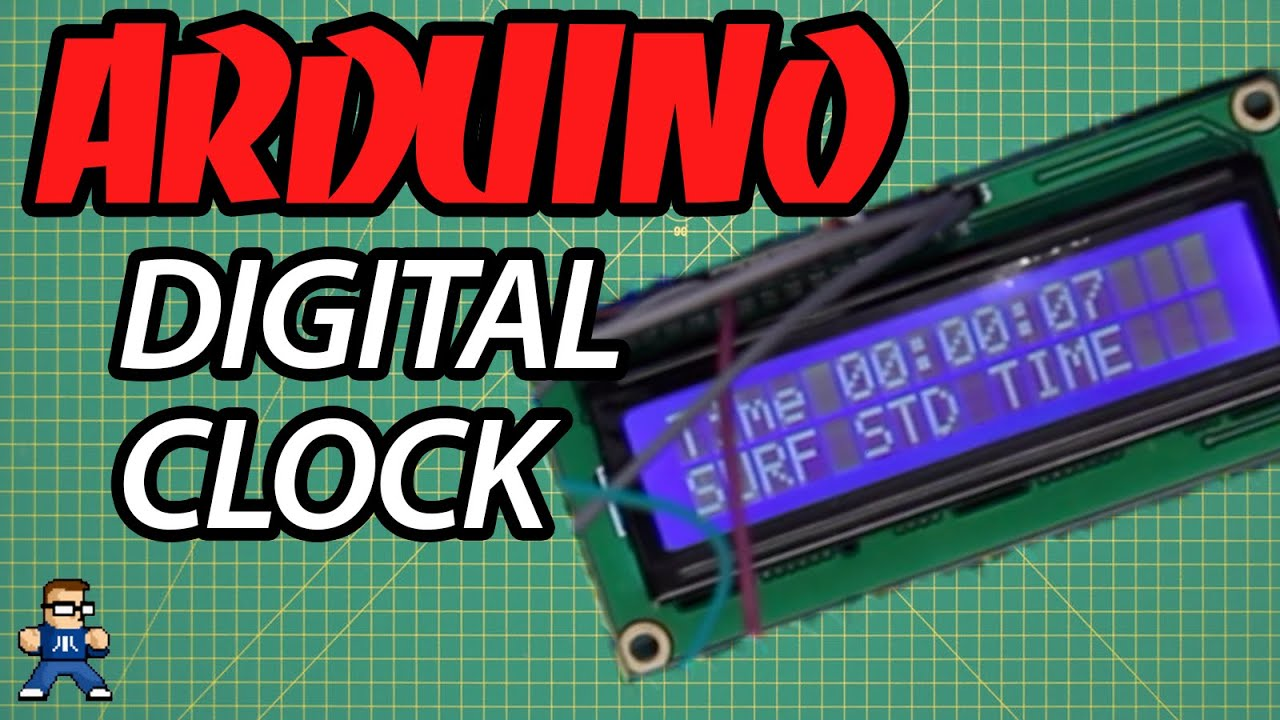# How To Build An Arduino Clock

Clocks are a rite of passage for hardware hackers, and with this video you can start working on your DIY Clock merit badge using the Arduino platform to build a basic Arduino clock. This project builds on the Arduino Fortune Teller project from the “Arduino For Kooks” basic series and teaches programming concepts like timing and actively updating a display, so you can use it as a springboard for many more complicated projects!

### PARTS/TOOLS:

(Most of these can be found in the Arduino Starter Kit available here)

Arduino UNO

1602 LCD

Assorted resistors (220 and 10K, specifically)

Jumper (Dupont) Wire

10K Potentiometer

Tactile switches

### The Circuit:Connect the LCD module as described in the Liquid Crystal Ball project. You can use the breadboard to create buses for +5V and GND. Connect one side of one tactile switch to D8 and the other to a 10K resistor to ground. Connect one side of the other tactile switch to D9 and the other side to a 10K resistor to ground.### The Sketch:

```//Projet ColorTyme //Phase 1: Simple LCD Clock //CC-BY-SA Matthew Eargle //AirborneSurfer.com //element14 Presents```

`#include "LiquidCrystal.h"`

```// Define LCD pins LiquidCrystal lcd(12, 11, 5, 4, 3, 2);```

```// initial Time display is 00:00:00 (24hr clock) int h=00; int m=00; int s=00;```

```// Time Set Buttons int button1; int button2; int hs=8;// pin 8 for Hours Setting int ms=9;// pin 9 for Minutes Setting```

```// Digital LCD Constrast setting int cs=6;// pin 5 for contrast PWM static int contrast=100;// default contrast```

```//Define current time as zero static uint32_t last_time, now = 0;```

```void setup() { lcd.begin(16,2); pinMode(hs,INPUT_PULLUP); pinMode(ms,INPUT_PULLUP);```

```now=millis(); // read RTC initial value analogWrite(cs,contrast); }```

```void loop() { // Update LCD Display // Print TIME in Hour, Min, Sec lcd.setCursor(0,0); lcd.print("Time "); if(h<10)lcd.print("0");// always 2 digits lcd.print(h); lcd.print(":"); if(m<10)lcd.print("0"); lcd.print(m); lcd.print(":"); if(s<10)lcd.print("0"); lcd.print(s);```
``` lcd.setCursor(0,1);// for Line 2 lcd.print("SURF STD TIME");```

```while ((now-last_time) < 1000 ) // wait1000ms { now=millis(); }```

```last_time=now; // prepare for next loop s=s+1; //increment sec. counting```
``` /-------Time setting-------/ button1=digitalRead(hs); if(button1==0) { s=0; h=h+1; }```

```button2=digitalRead(ms); if(button2==0){ s=0; m=m+1; }```

`analogWrite(cs,contrast); // update contrast`

```/* ---- manage seconds, minutes, hours am/pm overflow ----*/ if(s==60){ s=0; m=m+1; } if(m==60) { m=0; h=h+1; } if (h==25) { h=0; }```

`}`

## One thought on “How To Build An Arduino Clock”

This site uses Akismet to reduce spam. Learn how your comment data is processed.